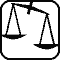Mathematical and Physical Journal
for High Schools
Issued by the MATFUND Foundation
 Already signed up? New to KöMaL?

# Exercises and problems in PhysicsFebruary 2005## Experimental problem

M. 258. A grease- or oil-spot becomes unnoticeable on a sheet of paper if the illumination of the two sides of the sheet is balanced out. Examine the illumination by a given light source (a torch for example) as a function of the distance and the angle of incidence.

(6 points)## Theoretical problems

It is allowed to send solutions for any number of problems, but final scores of students of grades 9-12 are computed from the 5 best score in each month. Final scores of students of grades 1-8 are computed from the 3 best scores in each month.

P. 3772. We throw three ice cubicles of a temperature of 0 oC into a glass of 18 oC water. The volume of the water is 2 dl. What is the volume and the temperature of the water after the ice cubes of an edge length of 2 cm have melted?

(3 points)

P. 3773. The table below shows the measured coefficient of specific heat at constant volume and the molar mass of some gases:

 H2 N2 O2 HCl cV (J/kg K) 10 112 741 653 573 M (g/mol) 2 28 32 36,5

a) What principle can be discerned from these measurement data? b) Is this principle in accordance with the taught theory?

(4 points)

P. 3774. Two slopes of angles of 30o are turned face-to-face with each other with their lower edges making contact. Determine all the points of the plane perpendicular to both slopes a small ball released from which its motion becomes periodic with four bounces in one cycle. (Collisions are perfectly elastic and friction is negligible.)

(5 points)

P. 3775. A solid ball rolls off the top of a fixed hemisphere having a rugged surface. Where does it begin to slip? What is the friction force at the moment of the first slip if $\displaystyle \mu$=$\displaystyle \mu$0=0.2; R=1 m; r=0.1 m; m=0.2 kg?

(5 points)

P. 3776. There is a smooth edged coin resting on a horizontal table top. Another coin of the same kind bumps into it. The collision coefficient is 0.8 here. At what angle can the moving coin be deflected from its original course at the most if neither of the coins gets a spin in the collision?

(5 points)

P. 3777. There is an insulator rod of length L and of negligible mass with two small balls of mass m and electric charge Q attached to its ends. The rod can rotate in the horizontal plane around a vertical axis crossing it at a L/4 distance from one of its ends. At first the rod is in instable equilibrium in a horizontal electric field of field strength E. Then we displace it from this position and leave it alone. a) Determine the maximum velocity of the ball closer to the axis. b) In what position is the rod to be set so that displaced from that position it begins a harmonic oscillation, and what is the period in this case?

(4 points)

P. 3778. Initially there is I0 electric current in a resistance connected to a power source of U0 open-circuit voltage. The power output - let us consider it linearly changing - decreases to zero in T time. a) What is the overall energy output of the power source of internal resistance Ri? b) In what time does the open-circuit voltage decrease to 75%? The internal and external resistances can be considered constant.

(4 points)

P. 3779. How much energy is needed to remove an alpha-particle from a Uranium-238 nucleus? What conclusion can be drawn from the value obtained? (Detailed atomic mass tables can be found on the internet, on pages such as http://ie.lbl.gov/toi2003/MassSearch.asp.)

(4 points)

P. 3780. In a sinusoidal electromagnetic wave propagating in air the amplitude of the electric field strength is $\displaystyle 0.06~\frac{\rm V}{\rm m}$, and its frequency is 300 MHz. a) What is the amplitude and wavelength of the magnetic induction vector in this wave? b) In what way would the answer change if these values belonged to an electromagnetic wave propagating in water instead of air? (At this frequency the relative dielectric constant of water is 81 and its magnetic permeability is 1 in good approximation.)

(5 points)

P. 3781. Prolonged observations of a binary star lead to the following conclusions: The duration of a revolution is 80 terrestrial years, the distance of the primary and the secondary stars is 23.12 astronomical unit. We can suppose that both stars revolve on a circular orbit and the ratio of the radii is 0.848. What is the mass of the stars expressed in Solar masses?

(4 points)